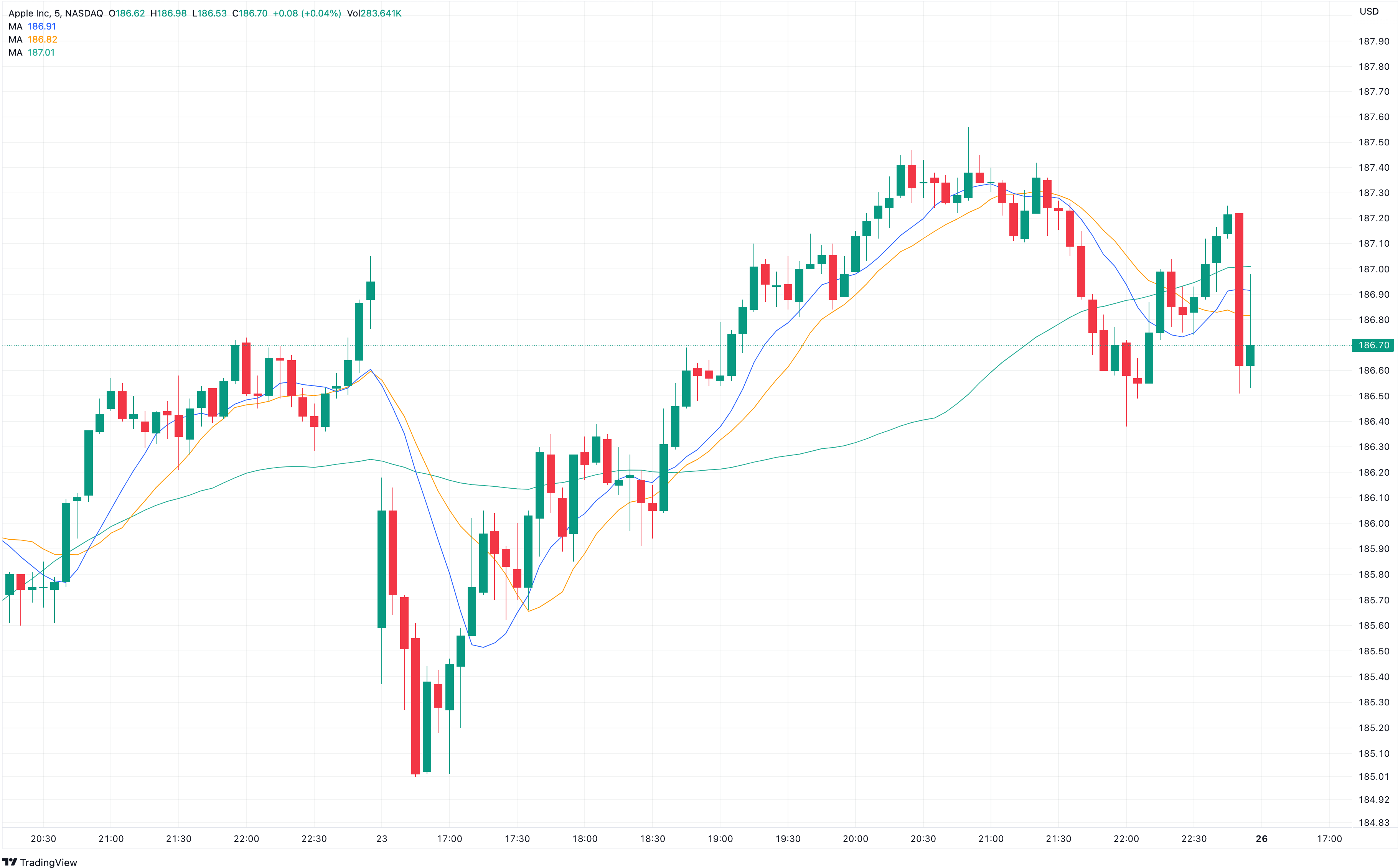# Understanding Moving Averages, MACD, RSI, and Stochastic Indicators in Technical Analysis

## Moving AveragesMoving averages (MAs) are used to smooth out price data and identify trends. They provide a clear picture of the overall direction of a market. The two most commonly used types of moving averages are the simple moving average (SMA) and the exponential moving average (EMA).

• Simple Moving Average (SMA): The SMA calculates the average price over a specific period by adding up the closing prices and dividing the sum by the number of periods. For example, a 50-day SMA calculates the average closing price over the past 50 days. Traders use SMAs to identify support and resistance levels and potential trend reversals when the price crosses above or below the moving average.

• Exponential Moving Average (EMA): The EMA places more weight on recent prices, making it more responsive to changes in price momentum compared to the SMA. The formula for calculating the EMA incorporates a smoothing factor that determines the weight given to each data point. The most commonly used EMA periods are 12 and 26, which are often used in conjunction with the MACD indicator (explained later). Traders use EMAs to identify shorter-term trends and generate trading signals when EMAs of different periods cross over.

Moving averages are versatile and can be applied to various timeframes. Shorter-term moving averages (e.g., 10 or 20 periods) react quickly to price changes, while longer-term moving averages (e.g., 50 or 200 periods) provide a broader view of the market trend.